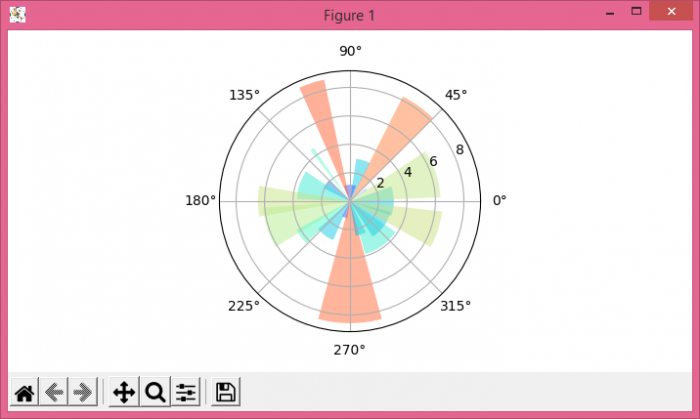# Circular (polar) histogram in Python

PythonMatplotlibServer Side ProgrammingProgramming

#### Beyond Basic Programming - Intermediate Python

Most Popular

36 Lectures 3 hours

#### Practical Machine Learning using Python

Best Seller

91 Lectures 23.5 hours

#### Practical Data Science using Python

22 Lectures 6 hours

To plot circular (polar) histogram in Python, we can take the following steps−

• Create data points for theta, radii and width using numpy.
• Add a subplot to the current figure, where projection='polar' and nrows=1, ncols=1 and index=1.
• . Make a bar plot using bar() method, with theta, radii and width data points
• Iterate radii and bars after zipping them together and set the face color of the bar and the alpha value. Lesser the alpha value, greater the transparency.
• To display the figure, use show() method.

## Example

import numpy as np
import matplotlib.pyplot as plt
plt.rcParams["figure.figsize"] = [7.00, 3.50]
plt.rcParams["figure.autolayout"] = True
N = 20
theta = np.linspace(0.0, 2 * np.pi, N, endpoint=False)
radii = 10 * np.random.rand(N)
width = np.pi / 4 * np.random.rand(N)
ax = plt.subplot(111, projection='polar')
bars = ax.bar(theta, radii, width=width, bottom=0.0)
for r, bar in zip(radii, bars):
bar.set_facecolor(plt.cm.rainbow(r / 10.0))
bar.set_alpha(0.5)
plt.show()

## Output